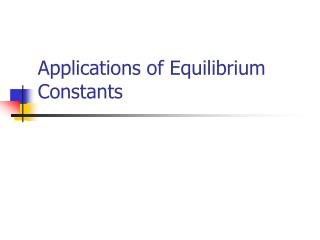DownloadDownload PresentationApplications of Equilibrium Constants

# Applications of Equilibrium Constants

Télécharger la présentation## Applications of Equilibrium Constants

- - - - - - - - - - - - - - - - - - - - - - - - - - - E N D - - - - - - - - - - - - - - - - - - - - - - - - - - -
##### Presentation Transcript

1. Applications of Equilibrium Constants

2. Example For the reaction below 2A + 3B  2C A 1.5L container is initially charged with 2.3 mole of A and 3.0 mole of B. At equilibrium, .75 mole of A is present. Calculate the value of the equilibrium constant.

3. Example • For the reaction PCl5 PCl3 + Cl2 the equilibrium constant Kp is .497. A cylinder is charged with PCl5 at an initial pressure of 2.20 atm. What are the equilibrium pressures of all of the substances?

4. Predicting Reaction Direction • If a mixture of reactants and products is not at equilibrium, we can use the equilibrium constant to predict whether the reaction will proceed to the right or left

5. Reaction Quotient (Q) • When current concentrations of products and reactants are substituted into the equilibrium constant expression • Q > K proceeds left (to reactant) • Q = K in equilibrium • Q < K proceeds right (to product)

6. Example • For the Haber process below, we have found the Kc to be .105. If we place 2.00M H2, 1.00M N2, and 2.00M NH3 in the same container, will the reaction proceed to the right or left? N2 + 3H2 2NH3

7. Example • At 448°C the equilibrium constant for the reaction H2 + I2 2HI is 50.5. Predict the direction in which the reaction will proceed to reach equilibrium if we start with .020 mol of HI, .010 mol of H2, and .030 mol of I2 in a 2.0 L container.